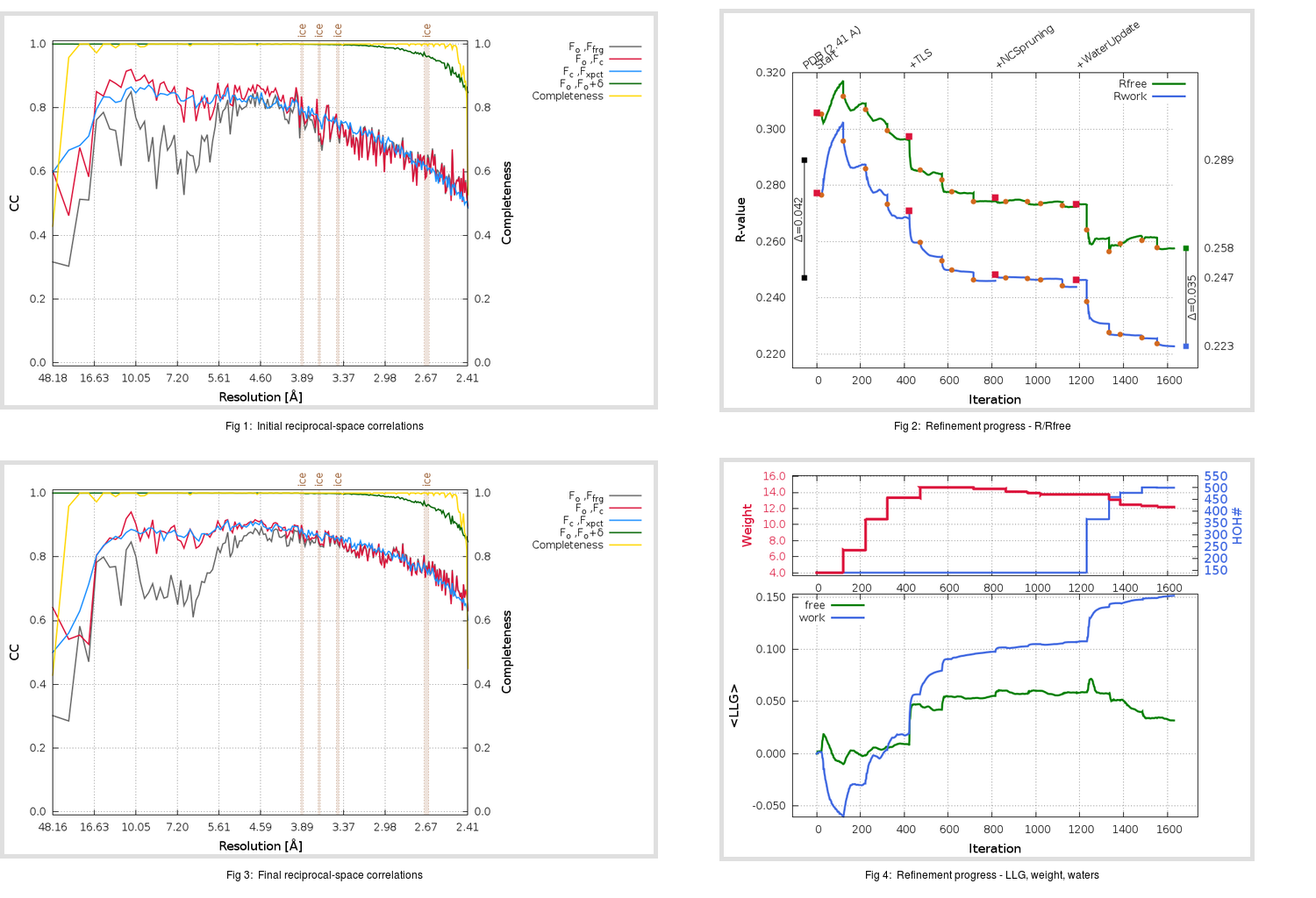Content:

```    Diffraction limits & principal axes of ellipsoid fitted to diffraction cut-off surface:
2.363         1.0000   0.0000   0.0000       a*
2.493         0.0000   1.0000   0.0000       b*
2.356         0.0000   0.0000   1.0000       c*
```

## Deposited

` `
 Date deposited Date data collection Resolution R, Rfree 20150910 20141130 2.41 0.2450 0.2890

Molprobity (CCP4 7.0 version) summary:

```Ramachandran outliers =   0.79 %
favored =  95.15 %
Rotamer outliers      =   5.01 %
C-beta deviations     =     0
Clashscore            =  13.44
RMS(bonds)            =   0.0041
RMS(angles)           =   0.95
MolProbity score      =   2.51
Resolution            =   2.41
R-work                =   0.2450
R-free                =   0.2890
```

```Number of waters      =   139

<B> (all atoms) =   73.88 ( sd =   39.11 ) for       9937 non-hydrogen atoms
<B>   (protein) =   74.08 ( sd =   39.33 ) for       9784 non-hydrogen atoms
<B>     (water) =   58.86 ( sd =   16.12 ) for        139 non-hydrogen atoms
<B>    (others) =   81.43 ( sd =    0.01 ) for         14 non-hydrogen atoms

B min/max       (all non-hydrogen atoms) =   25.10 /  246.29
B min/max   (protein non-hydrogen atoms) =   25.10 /  246.29
B min/max     (water non-hydrogen atoms) =   27.89 /   73.59
B min/max     (other non-hydrogen atoms) =   81.42 /   81.44
```

## BUSTER (re-)refinement

` `

Molprobity (CCP4 7.0 version) summary:

```Ramachandran outliers =   0.56 %
favored =  96.82 %
Rotamer outliers      =   5.80 %
C-beta deviations     =     8
Clashscore            =   4.36
RMS(bonds)            =   0.0112
RMS(angles)           =   1.63
MolProbity score      =   1.99
Resolution            =   2.41
R-work                =   0.2227
R-free                =   0.2576
```

```Number of waters      =   500

<B> (all atoms) =   72.74 ( sd =   36.96 ) for      10298 non-hydrogen atoms
<B>   (protein) =   73.75 ( sd =   37.51 ) for       9784 non-hydrogen atoms
<B>     (water) =   52.50 ( sd =   12.99 ) for        500 non-hydrogen atoms
<B>    (others) =   85.38 ( sd =    0.71 ) for         14 non-hydrogen atoms

B min/max       (all non-hydrogen atoms) =   22.96 /  193.06
B min/max   (protein non-hydrogen atoms) =   22.96 /  193.06
B min/max     (water non-hydrogen atoms) =   26.37 /  130.41
B min/max     (other non-hydrogen atoms) =   84.04 /   86.32
```

Refinement progression:Results:

` `
 File Remark 5DO2_aB_refine.01_04_refine.pdb.gz exact refinement commands are in header 5DO2_aB_refine.01_04_refine.mtz.gz including original deposited data and several re-refinement map coefficients 5DO2_aB_refine.01_04_BUSTER_model.cif.gz including any non-standard compound restraints 5DO2_aB_refine.01_04_BUSTER_refln.cif.gz